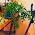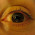MATH 1280 Introduction to Statistics – Discussion Unit 4 – Part 2

Madonna and child, painted by Sandro Botticelli

3 keywords in this discussion are random variables, the sample space and probability. Random variable is a function that goes from a sample space to a measurable space.

Sample space is part of a probability space. A sample space is a set of all the possible "outcomes" that may arise in an experiment.

Now, we make example to understand the concept. Let’s say that we have a coin. The coin has Head=H and Tail=T.

Then, we flipped the coin 4 times. The possible outcomes are: 2 and trials are 4.

We determine Sample Space = S = 2^4 = 16 = { HHHH, HTHH, THHH, HTHT, HHHT, HTTH, TTHH, THTH, HHTT, HHTH, TTTH, THHT, HTTT, TTTT, TTHT, THTT}

Virgin, child and angels, painted by Sandro Botticelli

Let’s say we just interest on the number of heads in four flips:
x = number of heads in four flips of a coin.
The random variable x could equal 0, 1, 2, 3, or 4.

We can determine the probability of x values by computing:
x= 0 = p(0)= probability no head = 1/16 = 0.0625
x = 1 = p(1)= probability 1 head = 4/16 = 0.25
x = 2 = p (2)= probability 2 heads = 6/16 = 0.375
x = 3 = p (3)= probability 3 heads = 4/16 = 0.25
x = 4 = p (4)= probability 4 heads = 1/16= 0.0625

By following this concept, we can determine the probability of tails too.

Finally, the sum of all the probabilities is one (1). We can prove it by adding probability of x values that is : 0.0625 + 0.25 + 0.375 + 0.25+ 0.0625 = 1

1.you know I never understood this stuff when I was in school and now it is even farther from my understanding

1.Thank you to visit:
MATH 1280 Introduction to Statistics – Discussion Unit 4 – Part 2
-
Madonna and child, painted by Sandro Botticelli

2.I enjoy the classic artwork.

1.Thank you to visit:
MATH 1280 Introduction to Statistics – Discussion Unit 4 – Part 2
-
Answer for: MATH 1280 Introduction to Statistics – Discussion Unit 4 – Part 1

3.This is absolutely mind boggling! Thanks for sharing such interesting information :)

1.Thank you to visit:
MATH 1280 Introduction to Statistics – Discussion Unit 4 – Part 2
-
3 keywords in this discussion are random variables, the sample space and probability. Random variable is a function that goes from a sample space to a measurable space.

4.Duh saya jadi pusing mas hehehe. Matematika dan probabilitas sudah lama saya tinggalkan :)

1.Thank you to visit:
MATH 1280 Introduction to Statistics – Discussion Unit 4 – Part 2
-
Sample space is part of a probability space. A sample space is a set of all the possible "outcomes" that may arise in an experiment.

5.You've got to hand to Botticelli.

1.Thank you to visit:
MATH 1280 Introduction to Statistics – Discussion Unit 4 – Part 2
-
Now, we make example to understand the concept. Let’s say that we have a coin. The coin has Head=H and Tail=T.

6.I think I will never understand that tricks :)

1.Thank you to visit:
MATH 1280 Introduction to Statistics – Discussion Unit 4 – Part 2
-
Then, we flipped the coin 4 times. The possible outcomes are: 2 and trials are 4.

7.Thanks for sharing. :-)

Best regards

1.Thank you to visit:
MATH 1280 Introduction to Statistics – Discussion Unit 4 – Part 2
-
We determine Sample Space = S = 2^4 = 16 = { HHHH, HTHH, THHH, HTHT, HHHT, HTTH, TTHH, THTH, HHTT, HHTH, TTTH, THHT, HTTT, TTTT, TTHT, THTT}

8.It's a great number trick :) I like so much this all paintings :-)

1.Thank you to visit:
MATH 1280 Introduction to Statistics – Discussion Unit 4 – Part 2
-
Virgin, child and angels, painted by Sandro Botticelli

9.I am surprised that I passed my Statistics class.

1.Thank you to visit:
MATH 1280 Introduction to Statistics – Discussion Unit 4 – Part 2
-
Let’s say we just interest on the number of heads in four flips:
x = number of heads in four flips of a coin.
The random variable x could equal 0, 1, 2, 3, or 4.

10.Interesting post.
I wish you a nice day.

1.Thank you to visit:
MATH 1280 Introduction to Statistics – Discussion Unit 4 – Part 2
-
We can determine the probability of x values by computing:
x= 0 = p(0)= probability no head = 1/16 = 0.0625
x = 1 = p(1)= probability 1 head = 4/16 = 0.25
x = 2 = p (2)= probability 2 heads = 6/16 = 0.375
x = 3 = p (3)= probability 3 heads = 4/16 = 0.25
x = 4 = p (4)= probability 4 heads = 1/16= 0.0625

11.Another very good statistics post! :)
--
O diário da Inês | Facebook | Instagram

1.Thank you to visit:
MATH 1280 Introduction to Statistics – Discussion Unit 4 – Part 2
-

12.Thank you for this post :)

1.Thank you to visit:
MATH 1280 Introduction to Statistics – Discussion Unit 4 – Part 2
-
By following this concept, we can determine the probability of tails too.

13.thanks for sharing!

1.Thank you to visit:
MATH 1280 Introduction to Statistics – Discussion Unit 4 – Part 2
-
Finally, the sum of all the probabilities is one (1). We can prove it by adding probability of x values that is : 0.0625 + 0.25 + 0.375 + 0.25+ 0.0625 = 1

14.This rings a bell... I totally studied this in school, way back.

1.Thank you to visit:
MATH 1280 Introduction to Statistics – Discussion Unit 4 – Part 2
-
Madonna and child, painted by Sandro Botticelli

15.I don't understand the maths, but the pictures are beautiful!

1.Thank you to visit:
MATH 1280 Introduction to Statistics – Discussion Unit 4 – Part 2
-
Answer for: MATH 1280 Introduction to Statistics – Discussion Unit 4 – Part 1

16.Thanks for sharing. I did study this in school, though can't remember much now!

1.Thank you to visit:
MATH 1280 Introduction to Statistics – Discussion Unit 4 – Part 2
-
3 keywords in this discussion are random variables, the sample space and probability. Random variable is a function that goes from a sample space to a measurable space.

17.Oh no, I am not a big fun of the math.

1.Thank you to visit:
MATH 1280 Introduction to Statistics – Discussion Unit 4 – Part 2
-
Sample space is part of a probability space. A sample space is a set of all the possible "outcomes" that may arise in an experiment.